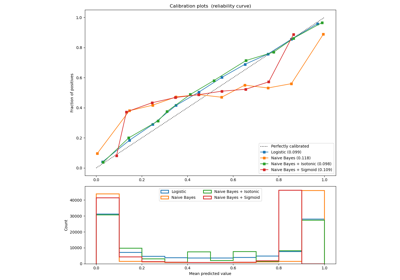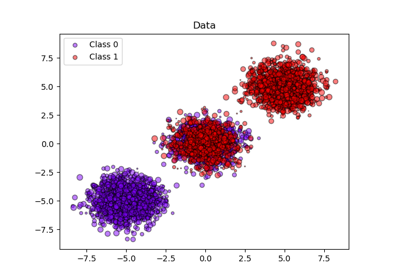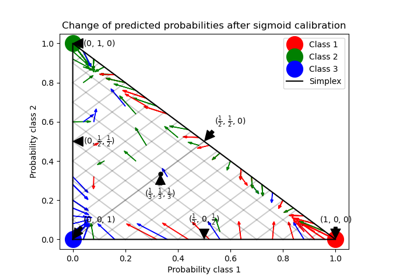# sklearn.calibration.CalibratedClassifierCV¶

class sklearn.calibration.CalibratedClassifierCV(base_estimator=None, *, method='sigmoid', cv=None)[source]

Probability calibration with isotonic regression or logistic regression.

The calibration is based on the decision_function method of the base_estimator if it exists, else on predict_proba.

Read more in the User Guide.

Parameters
base_estimatorinstance BaseEstimator

The classifier whose output need to be calibrated to provide more accurate predict_proba outputs.

method‘sigmoid’ or ‘isotonic’

The method to use for calibration. Can be ‘sigmoid’ which corresponds to Platt’s method (i.e. a logistic regression model) or ‘isotonic’ which is a non-parametric approach. It is not advised to use isotonic calibration with too few calibration samples (<<1000) since it tends to overfit.

cvinteger, cross-validation generator, iterable or “prefit”, optional

Determines the cross-validation splitting strategy. Possible inputs for cv are:

• None, to use the default 5-fold cross-validation,

• integer, to specify the number of folds.

• An iterable yielding (train, test) splits as arrays of indices.

For integer/None inputs, if y is binary or multiclass, sklearn.model_selection.StratifiedKFold is used. If y is neither binary nor multiclass, sklearn.model_selection.KFold is used.

Refer User Guide for the various cross-validation strategies that can be used here.

If “prefit” is passed, it is assumed that base_estimator has been fitted already and all data is used for calibration.

Changed in version 0.22: cv default value if None changed from 3-fold to 5-fold.

Attributes
classes_array, shape (n_classes)

The class labels.

calibrated_classifiers_list (len() equal to cv or 1 if cv == “prefit”)

The list of calibrated classifiers, one for each cross-validation fold, which has been fitted on all but the validation fold and calibrated on the validation fold.

References

1

Obtaining calibrated probability estimates from decision trees and naive Bayesian classifiers, B. Zadrozny & C. Elkan, ICML 2001

2

Transforming Classifier Scores into Accurate Multiclass Probability Estimates, B. Zadrozny & C. Elkan, (KDD 2002)

3

Probabilistic Outputs for Support Vector Machines and Comparisons to Regularized Likelihood Methods, J. Platt, (1999)

4

Predicting Good Probabilities with Supervised Learning, A. Niculescu-Mizil & R. Caruana, ICML 2005

Methods

 fit(X, y[, sample_weight]) Fit the calibrated model get_params([deep]) Get parameters for this estimator. Predict the target of new samples. Posterior probabilities of classification score(X, y[, sample_weight]) Return the mean accuracy on the given test data and labels. set_params(**params) Set the parameters of this estimator.
__init__(base_estimator=None, *, method='sigmoid', cv=None)[source]

Initialize self. See help(type(self)) for accurate signature.

fit(X, y, sample_weight=None)[source]

Fit the calibrated model

Parameters
Xarray-like, shape (n_samples, n_features)

Training data.

yarray-like, shape (n_samples,)

Target values.

sample_weightarray-like of shape (n_samples,), default=None

Sample weights. If None, then samples are equally weighted.

Returns
selfobject

Returns an instance of self.

get_params(deep=True)[source]

Get parameters for this estimator.

Parameters
deepbool, default=True

If True, will return the parameters for this estimator and contained subobjects that are estimators.

Returns
paramsmapping of string to any

Parameter names mapped to their values.

predict(X)[source]

Predict the target of new samples. The predicted class is the class that has the highest probability, and can thus be different from the prediction of the uncalibrated classifier.

Parameters
Xarray-like, shape (n_samples, n_features)

The samples.

Returns
Carray, shape (n_samples,)

The predicted class.

predict_proba(X)[source]

Posterior probabilities of classification

This function returns posterior probabilities of classification according to each class on an array of test vectors X.

Parameters
Xarray-like, shape (n_samples, n_features)

The samples.

Returns
Carray, shape (n_samples, n_classes)

The predicted probas.

score(X, y, sample_weight=None)[source]

Return the mean accuracy on the given test data and labels.

In multi-label classification, this is the subset accuracy which is a harsh metric since you require for each sample that each label set be correctly predicted.

Parameters
Xarray-like of shape (n_samples, n_features)

Test samples.

yarray-like of shape (n_samples,) or (n_samples, n_outputs)

True labels for X.

sample_weightarray-like of shape (n_samples,), default=None

Sample weights.

Returns
scorefloat

Mean accuracy of self.predict(X) wrt. y.

set_params(**params)[source]

Set the parameters of this estimator.

The method works on simple estimators as well as on nested objects (such as pipelines). The latter have parameters of the form <component>__<parameter> so that it’s possible to update each component of a nested object.

Parameters
**paramsdict

Estimator parameters.

Returns
selfobject

Estimator instance.

## Examples using sklearn.calibration.CalibratedClassifierCV¶